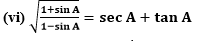# NCERT Solutions for Class 10 Maths Chapter 8 Introduction to Trigonometry (Exercise – 8.4)## Ex 8.4 Question 1.

Express the trigonometric ratios sin A, sec A and tan A in terms of cot A.

Solution:## Ex 8.4 Question 2.

Write all the other trigonometric ratios of ∠A in terms of sec A.

Solution:## Ex 8.4 Question 3.

Evaluate:

(i) (sin263° + sin227°)/(cos217° + cos273°)
(ii)  sin 25° cos 65° + cos 25° sin 65°

Solution:4. Choose the correct option. Justify your choice.
(i) 9 sec2A – 9 tan2A =
(A) 1                 (B) 9              (C) 8                (D) 0
(ii) (1 + tan θ + sec θ) (1 + cot θ – cosec θ)
(A) 0                 (B) 1              (C) 2                (D) – 1
(iii) (sec A + tan A) (1 – sin A) =
(A) sec A           (B) sin A        (C) cosec A      (D) cos A

(iv) 1+tan2A/1+cot2A =

(A) secA                 (B) -1              (C) cot2A                (D) tan2A

Solution:5. Prove the following identities, where the angles involved are acute angles for which the
expressions are defined.

(i) (cosec θ – cot θ)= (1-cos θ)/(1+cos θ)

(ii) cos A/(1+sin A) + (1+sin A)/cos A = 2 sec A

(iii) tan θ/(1-cot θ) + cot θ/(1-tan θ) = 1 + sec θ cosec θ

[Hint : Write the expression in terms of sin θ and cos θ]

(iv) (1 + sec A)/sec A = sin2A/(1-cos A)

[Hint : Simplify LHS and RHS separately]

(v) ( cos A–sin A+1)/( cos A +sin A–1) = cosec A + cot A, using the identity cosec2A = 1+cot2A.(vii) (sin θ – 2sin3θ)/(2cos3θ-cos θ) = tan θ
(viii) (sin A + cosec A)+ (cos A + sec A)2 = 7+tan2A+cot2A
(ix) (cosec A – sin A)(sec A – cos A) = 1/(tan A+cotA)
[Hint : Simplify LHS and RHS separately]
(x) (1+tan2A/1+cot2A) = (1-tan A/1-cot A)2 = tan2A

Solution:error: Content is protected !!